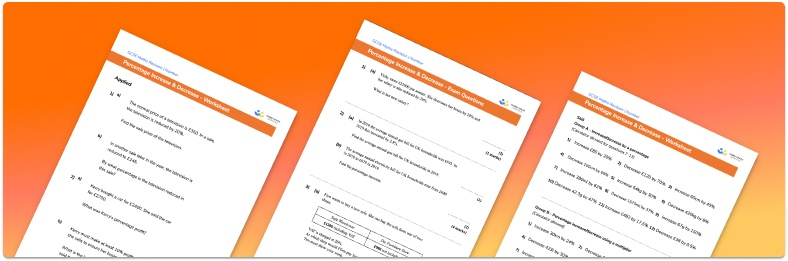# Percentage Increase And Decrease Worksheet• Section 1 of the percentage increase and decrease worksheet contains 36 skills-based percentage increase and decrease questions, in 3 groups to support differentiation
• Section 2 contains 4 applied percentage increase and decrease questions with a mix of word problems and deeper problem solving questions
• Section 3 contains 4 foundation and higher level GCSE percentage increase and decrease exam questions
• Answers and a mark scheme for all percentage increase and decrease practice questions are provided
• Percentage problems follow variation theory with plenty of opportunities for students to work independently at their own level
• All questions created by fully qualified expert secondary maths teachers

Suitable for GCSE maths revision for AQA, OCR and Edexcel exam boards

• This field is for validation purposes and should be left unchanged.

You can unsubscribe at any time (each email we send will contain an easy way to unsubscribe). To find out more about how we use your data, see our privacy policy.

### Percentage increase and decrease at a glance

Once students are confident with calculating percentages of amounts, we move to percentage increase and decrease. A simple starting point is a real-life scenario like a wage increase – for example, last year someone earned a wage of £25000, and received a 10% increase. To calculate their new salary in the next year, we find 10% of £25000 and add this on to the original amount. If it was a wage deduction, we use subtraction from the original value. For easier percentages and multiples of 10%, students may prefer this method.

If a calculator is available, the percentage multiplier method is often quicker – for example, finding the sale price of an item reduced by 35% with an original price of £40. Here, the multiplier is 0.65 (1 0.35), so work out 0.65 40 to find the new price of the item.

Extending this topic further, students study the reverse process where they are given the new number and the percentage change and need to  work backwards to find the original number.

Looking forward, students can then progress to additional number worksheets, for example a dividing fractions worksheet or a compound interest worksheet.For more teaching and learning support on Number our GCSE maths lessons provide step by step support for all GCSE maths concepts.

## Do you have KS4 students who need more focused attention to succeed at GCSE?There will be students in your class who require individual attention to help them succeed in their maths GCSEs. In a class of 30, it’s not always easy to provide.

Help your students feel confident with exam-style questions and the strategies they’ll need to answer them correctly with our dedicated GCSE maths revision programme.

Lessons are selected to provide support where each student needs it most, and specially-trained GCSE maths tutors adapt the pitch and pace of each lesson. This ensures a personalised revision programme that raises grades and boosts confidence.

Find out more